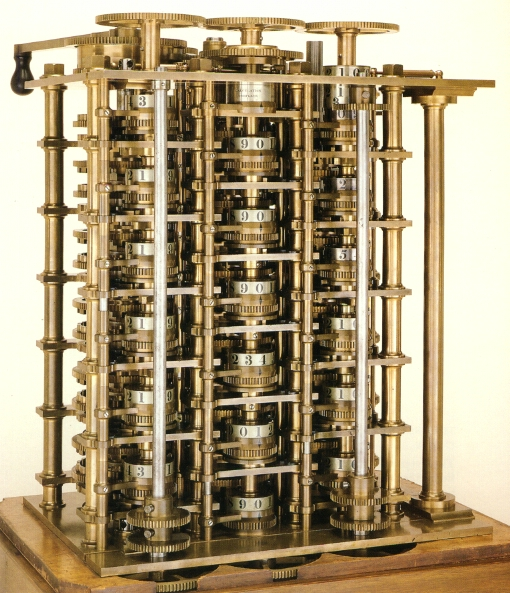Prior to the invention of the more famous and never realized Analytical Engine, Charles Babbage conceived of an engine based upon the principles of constant difference. He first thought of the idea for the Difference Engine in 1821, when remarking to his friend how prone to error and inaccurate existing tables of values were. Before the invention of computers, values such as sines, cosines, natural logs, etc. were stored in volumes of hand computed tables. These tables suffered from large amounts of inaccurate entries, but were necessary for practical fields such as navigation. Babbage intended to replace these volumes with tables printed by a machine incapable of being inaccurate with proper precaution, and inevitably quicker than manual computation. The principle of constant difference is an easy mathematical property to grasp. Consider the linear function f(x) = 2x + 2. Writing the first few values in a table, the result is: x: f(x): Difference (as expressed by f(x+1) – f(x)): 1 4 - 2 6 2 3 8 2 4 10 2 … … With each successive increment of x, the result increases by two. However, it may seem that very few functions have such an obvious constant difference. While many do not have a constant difference at the first order, there are different degrees of constant difference. Consider the quadratic function f(x) = x2. x: f(x) Difference 1: Difference 2: 1 1 - - 2 4 3 - 3 9 5 2 4 16 7 2 5 25 9 2 The first order of difference is not constant in this case, but the second is. As the degree of the exponential in an exponential function increases by 1, so does the amount of differences increasy by 1 before the constant difference is found. The engine itself consisted of 7 columns – 1 column to store the first value of the table to be calculated, and 6 columns to store the differences. Babbage considered 6 orders to be enough to do any useful calculation. It might be wondered at this point how polynomial equations might be useful. An example Babbage cited was for use at the London shipyards. They could use tables to quickly know how many cannon balls are required to put in a triangular stack n high. n: number of balls: Difference 1: Difference 2: Difference 3: 1 high : 1 ball. - - - 2 high: 4 balls. 3 - - 3 high: 10 balls. 6 3 - 4 high: 20 balls. 10 4 1 5 high: 35 balls. 15 5 1 Thus, at 3 orders of difference, there is a constant difference 1. Babbage was not content with polynomial equations alone however. He wanted to make a machine that could print sine tables, cosine tables, logarithms, and other functions that don’t have a constant difference. He proposed that by breaking these functions up over a variety of ranges, a constant difference can be found on a small level. Taking the sine function for example, 15 digit numbers with a 4th order constant difference can be used to accurately compute sine over a 2 degree angle. Thus, in order to find the sin values over a quadrant (90 degress), the machine would have to be reset 45 times. For clear reasons, this inefficient way of computing did not satisfy Babbage, and he jotted the idea of a machine not limited by differences down in his notes in 1822. In 1837, this idea was realized and the Analytical Engine was born.